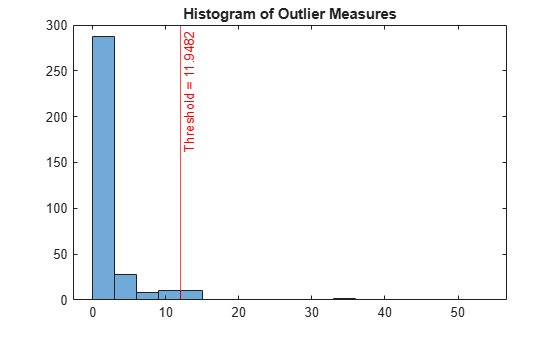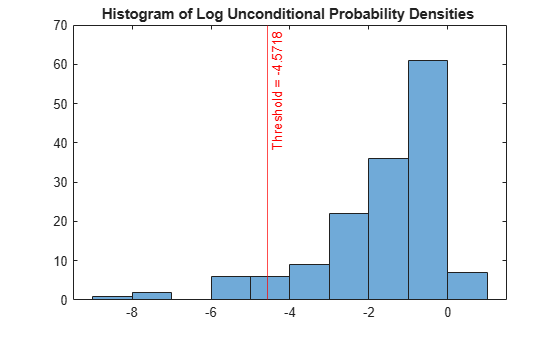Model-Specific Anomaly Detection

Statistics and Machine Learning Toolbox™ provides model-specific anomaly detection features that you can apply after training a classification, regression, or clustering model. For example, you can detect anomalies by using these object functions:

• Proximity matrix — outlierMeasure for random forest (CompactTreeBagger)

• Mahalanobis distance — mahal for discriminant analysis classifier (ClassificationDiscriminant) and mahal for Gaussian mixture model (gmdistribution)

• Unconditional probability density — logp for discriminant analysis classifier (ClassificationDiscriminant), logp for naive Bayes classifier (ClassificationNaiveBayes), and logp for naive Bayes classifier for incremental learning (incrementalClassificationNaiveBayes)

For details, see the function reference pages.

Detect Outliers After Training Random Forest

Train a random forest classifier by using the TreeBagger function, and detect outliers in the training data by using the object function outlierMeasure.

Train Random Forest Classifier

Load the ionosphere data set, which contains radar return qualities (Y) and predictor data (X) for 34 variables. Radar returns are either of good quality ('g') or bad quality ('b').

Train a random forest classifier. Store the out-of-bag information for predictor importance estimation.

rng("default") % For reproducibility
Mdl_TB = TreeBagger(100,X,Y,Method="classification", ...
OOBPredictorImportance="on");

Mdl_TB is a TreeBagger model object for classification. TreeBagger stores predictor importance estimates in the property OOBPermutedPredictorDeltaError.

Detect Outliers Using Proximity

Detect outliers in the training data by using the outlierMeasure function. The function computes outlier measures based on the average squared proximity between one observation and the other observations in the trained random forest.

CMdl_TB = compact(Mdl_TB);
s_proximity = outlierMeasure(CMdl_TB,X,Labels=Y);

A high value of the outlier measure indicates that the observation is an outlier. Find the threshold corresponding to the 95th percentile and identify outliers by using the isoutlier function.

[TF,~,U] = isoutlier(s_proximity,Percentiles=[0 95]);

Plot a histogram of the outlier measures. Create a vertical line at the outlier threshold.

histogram(s_proximity)
xline(U,"r-",join(["Threshold =" U]))
title("Histogram of Outlier Measures")Visualize observation values using the two most important features selected by the predictor importance estimates in the property OOBPermutedPredictorDeltaError.

[~,idx] = sort(Mdl_TB.OOBPermutedPredictorDeltaError,'descend');
TF_c = categorical(TF,[0 1],["Normal Points" "Anomalies"]);
gscatter(X(:,idx(1)),X(:,idx(2)),TF_c,"kr",".x",[],"on", ...
Mdl_TB.PredictorNames(idx(1)),Mdl_TB.PredictorNames(idx(2)))Train the classifier again without outliers, and plot the histogram of the outlier measures.

Mdl_TB = TreeBagger(100,X(~TF,:),Y(~TF),Method="classification");
s_proximity = outlierMeasure(CMdl_TB,X(~TF,:),Labels=Y(~TF));
histogram(s_proximity)
title("Histogram of Outlier Measures After Removing Outliers")Detect Outliers After Training Discriminant Analysis Classifier

Train a discriminant analysis model by using the fitcdiscr function, and detect outliers in the training data by using the object functions logp and mahal.

Train Discriminant Analysis Model

Load Fisher's iris data set. The matrix meas contains flower measurements for 150 different flowers. The variable species lists the species for each flower.

Train a discriminant analysis model using the entire data set.

Mdl = fitcdiscr(meas,species,PredictorNames= ...
["Sepal Length" "Sepal Width" "Petal Length" "Petal Width"]);

Mdl is a ClassificationDiscriminant model.

Detect Outliers Using Log Unconditional Probability Density

Compute the log unconditional probability densities of the training data.

s_logp = logp(Mdl,meas);

A low density value indicates that the corresponding observation is an outlier.

Determine the lower density threshold for outliers by using the isoutlier function.

[~,L_logp] = isoutlier(s_logp);

Identify outliers by using the threshold.

TF_logp = s_logp < L_logp;

Plot a histogram of the density values. Create a vertical line at the outlier threshold.

figure
histogram(s_logp)
xline(L_logp,"r-",join(["Threshold =" L_logp]))
title("Histogram of Log Unconditional Probability Densities ")To compare observation values between normal points and anomalies, create a matrix of grouped histograms and grouped scatter plots for each combination of variables by using the gplotmatrix function.

TF_logp_c = categorical(TF_logp,[0 1],["Normal Points" "Anomalies"]);
gplotmatrix(meas,[],TF_logp_c,"kr",".x",[],[],[],Mdl.PredictorNames)Detect Outliers Using Mahalanobis Distance

Find the squared Mahalanobis distances from the training data to the class means of true labels.

s_mahal = mahal(Mdl,meas,ClassLabels=species);

A large distance value indicates that the corresponding observation is an outlier.

Determine the threshold corresponding to the 95th percentile and identify outliers by using the isoutlier function.

[TF_mahal,~,U_mahal] = isoutlier(s_mahal,Percentiles=[0 95]);

Plot a histogram of the distances. Create a vertical line at the outlier threshold.

figure
histogram(s_mahal)
xline(U_mahal,"-r",join(["Threshold =" U_mahal]))
title("Histogram of Mahalanobis Distances")Compare the observation values between normal points and anomalies by using the gplotmatrix function.

TF_mahal_c = categorical(TF_mahal,[0 1],["Normal Points" "Anomalies"]);
gplotmatrix(meas,[],TF_mahal_c,"kr",".x",[],[],[],Mdl.PredictorNames)